# Setup for 4 Player a Game of Food Chain Magnate

Originally generated on 1/11/2019 7:21:09 AM

Like BoardGameHelpers.com? Then buy the microbadge
on BGG! If I see it displayed under your avatar, I'll refund you the 8gg!

Login to save this setup to My Games

## Player Setup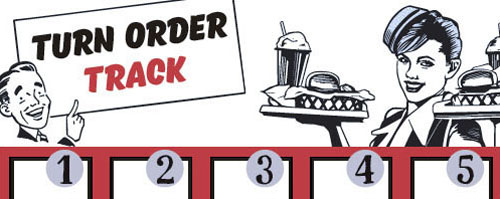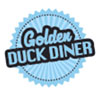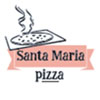## Other Setup

Bank starts with \$200.00
Remove these Billboards: #16
Number of 1x Employee cards used = 2

## Map (Random)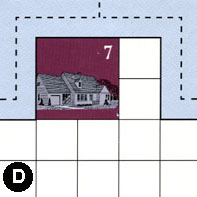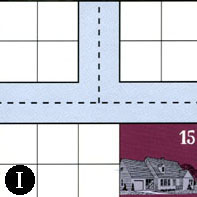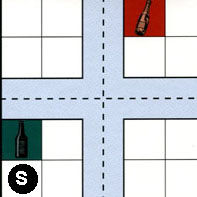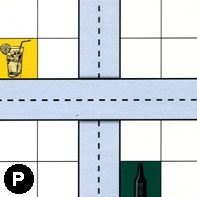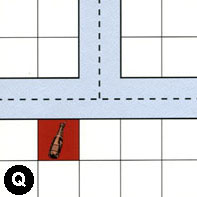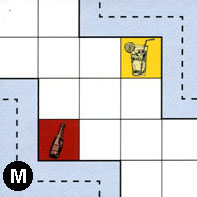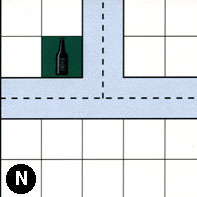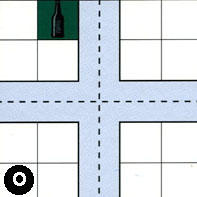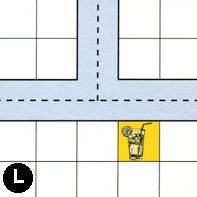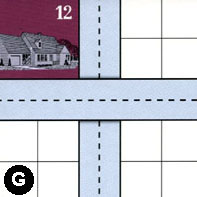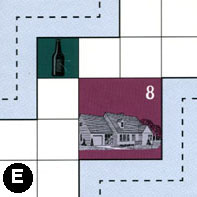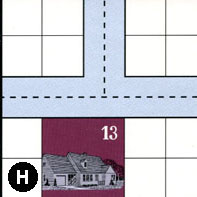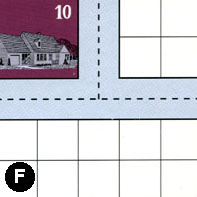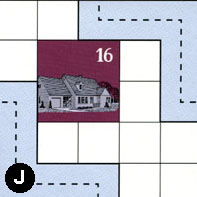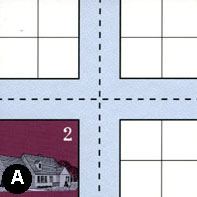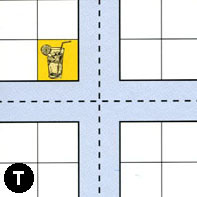### Map Stats

Map Option = Random
Total Number of Tiles = 16
Total Number of Starting Houses = 8
Total Number of Beer Spots = 5
Total Number of Soda Spots = 3
Total Number of Lemonade Spots = 4
Total Number of Drink Spots = 12
Number of independent path systems = 2
Number of independent neighborhoods = 17

Path System 1
Size = Medium
Number of Tiles = 14
Number of Paths = 48
Contains Loop(s) = No
Number of Starting Houses = 8
Starting Houses Pct of Map (%) = 100
Number of Beer Spots = 5
Number of Soda Spots = 3
Number of Lemonade Spots = 3
Number of Drink Spots = 11
Drink Spots Pct of Map (%) = 92
Tile Ids = A, D, E, G, H, I, J, M, N, O, P, Q, S, T
Paths = DWE, IWE, SWN, SNE, PNS, SWE, IWN, MEN, QWE, QWN, DWN, INE, DNE, QNE, SWS, NWN, OWN, PWE, ONE, OWE, NNE, EES, GWE, NWE, SNS, SES, OWS, HWN, EWN, AWS, TWN, TWE, TNE, AWN, ANE, JWS, GNS, MWS, AWE, TNS, TWS, TES, AES, ANS, HWE, HNE, ONS, OES What do these mean?
Starting House Ids = 0, 2, 7, 8, 12, 13, 15, 16
Path System 2
Size = Medium
Number of Tiles = 3
Number of Paths = 7
Contains Loop(s) = No
Number of Starting Houses = 3
Starting Houses Pct of Map (%) = 38
Number of Beer Spots = 0
Number of Soda Spots = 0
Number of Lemonade Spots = 1
Number of Drink Spots = 1
Drink Spots Pct of Map (%) = 8
Tile Ids = F, J, L
Paths = LWN, FWN, JEN, FNE, FWE, LWE, LNE What do these mean?
Starting House Ids = 0, 10, 16

Neighborhood 1
Total Size = Large
Number of Total Spaces = 30
Number of Empty Spaces (for new houses & gardens) = 22
Number of Beer Spots = 0
Number of Soda Spots = 0
Number of Lemonade Spots = 0
Number of Drink Spots = 0
Number of Starting Houses = 2
Starting Houses Pct of Map (%) = 25
Tile Ids = D, I, S
Starting House Ids = 7, 15
Neighborhood 2
Total Size = Medium
Number of Total Spaces = 8
Number of Empty Spaces (for new houses & gardens) = 7
Number of Beer Spots = 0
Number of Soda Spots = 1
Number of Lemonade Spots = 0
Number of Drink Spots = 1
Number of Starting Houses = 0
Starting Houses Pct of Map (%) = 0
Tile Ids = P, S
Neighborhood 3
Total Size = Small
Number of Total Spaces = 4
Number of Empty Spaces (for new houses & gardens) = 3
Number of Beer Spots = 1
Number of Soda Spots = 0
Number of Lemonade Spots = 0
Number of Drink Spots = 1
Number of Starting Houses = 0
Starting Houses Pct of Map (%) = 0
Tile Ids = P
Neighborhood 4
Total Size = Small
Number of Total Spaces = 4
Number of Empty Spaces (for new houses & gardens) = 4
Number of Beer Spots = 0
Number of Soda Spots = 0
Number of Lemonade Spots = 0
Number of Drink Spots = 0
Number of Starting Houses = 0
Starting Houses Pct of Map (%) = 0
Tile Ids = I
Neighborhood 5
Total Size = Large
Number of Total Spaces = 88
Number of Empty Spaces (for new houses & gardens) = 71
Number of Beer Spots = 1
Number of Soda Spots = 2
Number of Lemonade Spots = 2
Number of Drink Spots = 5
Number of Starting Houses = 3
Starting Houses Pct of Map (%) = 38
Tile Ids = A, F, G, I, J, L, M, N, Q, S
Starting House Ids = 10, 12, 16
Neighborhood 6
Total Size = Medium
Number of Total Spaces = 16
Number of Empty Spaces (for new houses & gardens) = 13
Number of Beer Spots = 2
Number of Soda Spots = 0
Number of Lemonade Spots = 1
Number of Drink Spots = 3
Number of Starting Houses = 0
Starting Houses Pct of Map (%) = 0
Tile Ids = N, O, P, S
Neighborhood 7
Total Size = Medium
Number of Total Spaces = 8
Number of Empty Spaces (for new houses & gardens) = 8
Number of Beer Spots = 0
Number of Soda Spots = 0
Number of Lemonade Spots = 0
Number of Drink Spots = 0
Number of Starting Houses = 0
Starting Houses Pct of Map (%) = 0
Tile Ids = O, P
Neighborhood 8
Total Size = Small
Number of Total Spaces = 4
Number of Empty Spaces (for new houses & gardens) = 4
Number of Beer Spots = 0
Number of Soda Spots = 0
Number of Lemonade Spots = 0
Number of Drink Spots = 0
Number of Starting Houses = 0
Starting Houses Pct of Map (%) = 0
Tile Ids = Q
Neighborhood 9
Total Size = Medium
Number of Total Spaces = 8
Number of Empty Spaces (for new houses & gardens) = 8
Number of Beer Spots = 0
Number of Soda Spots = 0
Number of Lemonade Spots = 0
Number of Drink Spots = 0
Number of Starting Houses = 0
Starting Houses Pct of Map (%) = 0
Tile Ids = I, Q
Neighborhood 10
Total Size = Large
Number of Total Spaces = 35
Number of Empty Spaces (for new houses & gardens) = 30
Number of Beer Spots = 1
Number of Soda Spots = 0
Number of Lemonade Spots = 0
Number of Drink Spots = 1
Number of Starting Houses = 1
Starting Houses Pct of Map (%) = 12
Tile Ids = A, E, G, H, N, O
Starting House Ids = 8
Neighborhood 11
Total Size = Medium
Number of Total Spaces = 8
Number of Empty Spaces (for new houses & gardens) = 8
Number of Beer Spots = 0
Number of Soda Spots = 0
Number of Lemonade Spots = 0
Number of Drink Spots = 0
Number of Starting Houses = 0
Starting Houses Pct of Map (%) = 0
Tile Ids = H, O
Neighborhood 12
Total Size = Large
Number of Total Spaces = 92
Number of Empty Spaces (for new houses & gardens) = 75
Number of Beer Spots = 1
Number of Soda Spots = 2
Number of Lemonade Spots = 2
Number of Drink Spots = 5
Number of Starting Houses = 3
Starting Houses Pct of Map (%) = 38
Tile Ids = A, F, G, I, J, L, M, N, Q, S
Starting House Ids = 10, 12, 16
Neighborhood 13
Total Size = Medium
Number of Total Spaces = 14
Number of Empty Spaces (for new houses & gardens) = 14
Number of Beer Spots = 0
Number of Soda Spots = 0
Number of Lemonade Spots = 0
Number of Drink Spots = 0
Number of Starting Houses = 0
Starting Houses Pct of Map (%) = 0
Tile Ids = F, L
Neighborhood 14
Total Size = Medium
Number of Total Spaces = 22
Number of Empty Spaces (for new houses & gardens) = 14
Number of Beer Spots = 0
Number of Soda Spots = 0
Number of Lemonade Spots = 0
Number of Drink Spots = 0
Number of Starting Houses = 2
Starting Houses Pct of Map (%) = 25
Tile Ids = A, H, T
Starting House Ids = 2, 13
Neighborhood 15
Total Size = Small
Number of Total Spaces = 4
Number of Empty Spaces (for new houses & gardens) = 4
Number of Beer Spots = 0
Number of Soda Spots = 0
Number of Lemonade Spots = 0
Number of Drink Spots = 0
Number of Starting Houses = 0
Starting Houses Pct of Map (%) = 0
Tile Ids = F
Neighborhood 16
Total Size = Medium
Number of Total Spaces = 8
Number of Empty Spaces (for new houses & gardens) = 7
Number of Beer Spots = 0
Number of Soda Spots = 0
Number of Lemonade Spots = 1
Number of Drink Spots = 1
Number of Starting Houses = 0
Starting Houses Pct of Map (%) = 0
Tile Ids = A, T
Neighborhood 17
Total Size = Small
Number of Total Spaces = 4
Number of Empty Spaces (for new houses & gardens) = 4
Number of Beer Spots = 0
Number of Soda Spots = 0
Number of Lemonade Spots = 0
Number of Drink Spots = 0
Number of Starting Houses = 0
Starting Houses Pct of Map (%) = 0
Tile Ids = T

v3.5.24